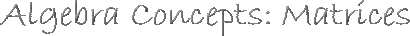Section 4.1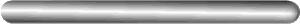4.1 Matrix Operations

4.2 Matrix Multiplication

4.3 Determinants

4.4 Identity and Inverse

4.5 Solving Systems Using Inverse Matrices

Real Life UsesSection 4.1: Exploring Data and Matrix Operations

To make it easier to identify matrices, we give them names based on
the number of rows and columns each one has.  When writing the names
of the matrices, use the number of the row first, then the number of
the column.
ex.  2 by 2.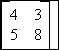Some general rules for naming matrices.
When a matrix has all elements zero, then it is called a zero matrix.
When a matrix has only one row it is called a row matrix.
When a matrix has only one column it is called a column matrix.
When the number of rows is equal to the number of columns, it is
called a square matrix.

You Try:
1)	This matrix is called a: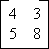2)	This matrix is called a: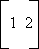3)	This matrix is called a: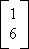1)	Square Matrix
2)	Row Matrix
3)	Column Matrix4.4 Identity and Inverse|4.5 Solving Systems Using Inverse Matrices

Real Life Uses